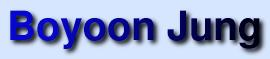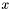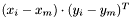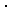Boyoon Jung's Library Manual

# bj::UnscentedKalmanFilter Class Reference [Kalman Filter]

An Unscented Kalman Filter class for non-linear systems. More...

#include <kalman.h>

List of all members.

## Public Member Functions

UnscentedKalmanFilter (int stateDim, int *measurementDim, int size, gsl_matrix *Rv=0, gsl_matrix **Rn=0)
A constructor.
UnscentedKalmanFilter (int stateDim, int measurementDim, gsl_matrix *Rv=0, gsl_matrix *Rn=0)
A constructor.
~UnscentedKalmanFilter (void)
A destructor.
void initialize (gsl_vector *x, gsl_matrix *P, gsl_matrix *Rv=0, gsl_matrix **Rn=0, int size=0)
Set parameters.
void initialize (gsl_vector *x, gsl_matrix *P, gsl_matrix *Rv, gsl_matrix *Rn)
Set parameters.
void timeUpdate (gsl_vector *u, gsl_matrix *Rv=0, double dt=0)
Evaluate the time update equation. [prediction step].
void timeUpdate (gsl_vector *u, double dt)
Evaluate the time update equation. [prediction step].
void measurementUpdate (gsl_vector *y, gsl_matrix *Rn=0, int index=0)
Evaluate the measurement update equation. [correction step].
void measurementUpdate (gsl_vector *y, int index)
Evaluate the measurement update equation. [correction step].
void update (gsl_vector *u, gsl_vector *y, gsl_matrix *Rv=0, gsl_matrix *Rn=0, int index=0, double dt=0)
Evaluate the time & the measurement update equations.
void update (gsl_vector *u, gsl_vector *y, int index, double dt)
Evaluate the time & the measurement update equations.
void update (gsl_vector *u, gsl_vector *y, int index)
Evaluate the time & the measurement update equations.
void update (gsl_vector *u, gsl_vector *y, double dt)
Evaluate the time & the measurement update equations.
gsl_vector * state (void)
Retrieve the current state estimate.
gsl_matrix * covariance (void)
Retrieve the current error covariance.
double alpha (void)
Retrieve the spread of sigma points.
void setAlpha (double a)
Set the spread of sigma points.
double beta (void)
Retrieve the incorporate prior knowledge of the distribution.
void setBeta (double b)
Set the incorporate prior knowledge of the distribution.
double kappa (void)
Retrieve the secondary scaling parameter.
void setKappa (double k)
Set the secondary scaling parameter.
void set (double a, double b, double k)
Set all parameters at once.

## Protected Member Functions

void gsl_matrix_inverse (gsl_matrix *org, gsl_matrix *inv, int index)
Compute the inverse matrix of a given square matrix.
void gsl_matrix_sqrt (gsl_matrix *in, gsl_matrix *out)
Compute the square root of a given covariance matrix.
void calc_weights (void)
Calculate weights.
void calc_weighted_vector_sum (gsl_vector *weights, gsl_matrix *vectors, gsl_vector *sum)
Calculate the sum of weighted vectors.
void calc_weighted_cov_sum (gsl_vector *weights, gsl_matrix *vectors, gsl_vector *mean, gsl_matrix *sum)
Calculate the sum of weighted covariance matrix.
void calc_weighted_cov_sum (gsl_vector *weights, gsl_matrix *vectors, gsl_vector *mean, gsl_matrix *sum, int index)
Calculate the sum of weighted covariance matrix.
void calc_weighted_cov_sum (gsl_vector *weights, gsl_matrix *vectors0, gsl_vector *mean0, gsl_matrix *vectors1, gsl_vector *mean1, gsl_matrix *sum, int index)
Calculate the sum of weighted covariance matrix.
void draw_sigma_points (gsl_vector *x, gsl_matrix *P, gsl_matrix *S)
Draw simga points.
virtual void f (gsl_vector *x, gsl_vector *u, gsl_vector *x_, double dt=0)=0
Define the nonlinear transition matrix function (in-place function).
virtual void h (gsl_vector *x_, gsl_vector *y, int index=0)=0
Define the nonlinear measurement matrix function.

## Static Protected Member Functions

double sqr (double d)
Compute the square of a floating-point number.
double fsqrt (double d)
Compute the square root of a floating-point number.

## Protected Attributes

int msize
The number of measurements.
double p_alpha
Spread of sigma points.
double p_beta
Incorporate prior knowledge of.
double p_kappa
Secondary scaling parameter.
int L
The dimension of the state.
double lambda
Composite scaling parameter.
double gamma
Another scaling parameter.
gsl_vector * Wm
Weights for mean.
gsl_vector * Wc
Weights for covariance.
int nPoints
The number of sigma points.
gsl_matrix * X
Sigma points.
gsl_matrix ** Y
Propagated sigma points.
gsl_vector * x
State estimate.
gsl_vector ** y
Measurement estimate.
gsl_matrix * P
Error covariance.
gsl_matrix * Rv
Process noise covariance.
gsl_matrix ** Rn
Measurement noise covariance.
gsl_matrix ** Pyy
Measurement estimate covariance.
gsl_matrix ** Pxy
Weighted sum of.
gsl_matrix ** K
Kalman gain.
gsl_permutation ** pi
int si
gsl_matrix ** itmm
gsl_eigen_symmv_workspace * w
gsl_vector * eval
gsl_matrix * evec
gsl_matrix * stnn
gsl_vector * tn
gsl_matrix * tnn
gsl_vector ** tm
gsl_matrix ** tnm
gsl_matrix ** tmm
gsl_vector_view vv0
gsl_vector_view vv1
gsl_matrix_view mv

## Detailed Description

An Unscented Kalman Filter class for non-linear systems.

This filter assumes an additive (zero mean) noise.

• Dynamic equations:• Noise distribution:• Abstract functions:
• f(x, u, x_, dt) := process function x_ = f(x, u, dt)
• h(x_, z) := measurement function z = h(x_)

## Constructor & Destructor Documentation

 bj::UnscentedKalmanFilter::UnscentedKalmanFilter ( int stateDim, int * measurementDim, int size, gsl_matrix * Rv = 0, gsl_matrix ** Rn = 0 )

A constructor.

This constructor is for when there are multiple measurements.

Parameters:
 stateDim The dimension of a state vector. measurementDim The dimensions of measurement vectors. size The number of measurements. Rv Covariance of process noise. Rn Covariance of measurement noise.

 bj::UnscentedKalmanFilter::UnscentedKalmanFilter ( int stateDim, int measurementDim, gsl_matrix * Rv = 0, gsl_matrix * Rn = 0 )

A constructor.

This constructor is for when there is a single measurement.

Parameters:
 stateDim The dimension of a state vector. measurementDim The dimension of a measurement vector. Rv Covariance of process noise. Rn Covariance of measurement noise.

 bj::UnscentedKalmanFilter::~UnscentedKalmanFilter ( void )
 A destructor.

## Member Function Documentation

 double bj::UnscentedKalmanFilter::alpha ( void )  [inline]
 Retrieve the spread of sigma points.

 double bj::UnscentedKalmanFilter::beta ( void )  [inline]
 Retrieve the incorporate prior knowledge of the distribution.

 void bj::UnscentedKalmanFilter::calc_weighted_cov_sum ( gsl_vector * weights, gsl_matrix * vectors0, gsl_vector * mean0, gsl_matrix * vectors1, gsl_vector * mean1, gsl_matrix * sum, int index )  [protected]
 Calculate the sum of weighted covariance matrix.

 void bj::UnscentedKalmanFilter::calc_weighted_cov_sum ( gsl_vector * weights, gsl_matrix * vectors, gsl_vector * mean, gsl_matrix * sum, int index )  [protected]
 Calculate the sum of weighted covariance matrix.

 void bj::UnscentedKalmanFilter::calc_weighted_cov_sum ( gsl_vector * weights, gsl_matrix * vectors, gsl_vector * mean, gsl_matrix * sum )  [protected]
 Calculate the sum of weighted covariance matrix.

 void bj::UnscentedKalmanFilter::calc_weighted_vector_sum ( gsl_vector * weights, gsl_matrix * vectors, gsl_vector * sum )  [protected]
 Calculate the sum of weighted vectors.

 void bj::UnscentedKalmanFilter::calc_weights ( void )  [protected]
 Calculate weights.

 gsl_matrix* bj::UnscentedKalmanFilter::covariance ( void )  [inline]
 Retrieve the current error covariance.

 void bj::UnscentedKalmanFilter::draw_sigma_points ( gsl_vector * x, gsl_matrix * P, gsl_matrix * S )  [protected]
 Draw simga points.

 virtual void bj::UnscentedKalmanFilter::f ( gsl_vector * x, gsl_vector * u, gsl_vector * x_, double dt = 0 )  [protected, pure virtual]
 Define the nonlinear transition matrix function (in-place function).

 double bj::UnscentedKalmanFilter::fsqrt ( double d )  [inline, static, protected]
 Compute the square root of a floating-point number.

 void bj::UnscentedKalmanFilter::gsl_matrix_inverse ( gsl_matrix * org, gsl_matrix * inv, int index )  [inline, protected]
 Compute the inverse matrix of a given square matrix.

 void bj::UnscentedKalmanFilter::gsl_matrix_sqrt ( gsl_matrix * in, gsl_matrix * out )  [inline, protected]
 Compute the square root of a given covariance matrix.

 virtual void bj::UnscentedKalmanFilter::h ( gsl_vector * x_, gsl_vector * y, int index = 0 )  [protected, pure virtual]
 Define the nonlinear measurement matrix function.

 void bj::UnscentedKalmanFilter::initialize ( gsl_vector * x, gsl_matrix * P, gsl_matrix * Rv, gsl_matrix * Rn )  [inline]

Set parameters.

This is for when there is a single measurement.

Parameters:
 x Initial state estimate. P Initial error covariance. Rv Covariance of process noise. Rn Covariance of measurement noise.

 void bj::UnscentedKalmanFilter::initialize ( gsl_vector * x, gsl_matrix * P, gsl_matrix * Rv = 0, gsl_matrix ** Rn = 0, int size = 0 )

Set parameters.

This is for when there are multiple measurements.

Parameters:
 x Initial state estimate. P Initial error covariance. Rv Covariance of process noise. Rn Covariance of measurement noise. size The number of measurements.

 double bj::UnscentedKalmanFilter::kappa ( void )  [inline]
 Retrieve the secondary scaling parameter.

 void bj::UnscentedKalmanFilter::measurementUpdate ( gsl_vector * y, int index )  [inline]

Evaluate the measurement update equation. [correction step].

Parameters:
 y Measurement. index The index of a measurement when there are multiple measurements available.

 void bj::UnscentedKalmanFilter::measurementUpdate ( gsl_vector * y, gsl_matrix * Rn = 0, int index = 0 )

Evaluate the measurement update equation. [correction step].

Parameters:
 y Measurement. Rn Covariance of measurement noise. index The index of a measurement when there are multiple measurements available.

 void bj::UnscentedKalmanFilter::set ( double a, double b, double k )  [inline]
 Set all parameters at once.

 void bj::UnscentedKalmanFilter::setAlpha ( double a )  [inline]
 Set the spread of sigma points.

 void bj::UnscentedKalmanFilter::setBeta ( double b )  [inline]
 Set the incorporate prior knowledge of the distribution.

 void bj::UnscentedKalmanFilter::setKappa ( double k )  [inline]
 Set the secondary scaling parameter.

 double bj::UnscentedKalmanFilter::sqr ( double d )  [inline, static, protected]
 Compute the square of a floating-point number.

 gsl_vector* bj::UnscentedKalmanFilter::state ( void )  [inline]
 Retrieve the current state estimate.

 void bj::UnscentedKalmanFilter::timeUpdate ( gsl_vector * u, double dt )  [inline]

Evaluate the time update equation. [prediction step].

Parameters:
 u Control input. dt Time interval.

 void bj::UnscentedKalmanFilter::timeUpdate ( gsl_vector * u, gsl_matrix * Rv = 0, double dt = 0 )

Evaluate the time update equation. [prediction step].

Parameters:
 u Control input. Rv Covariance of process noise. dt Time interval.

 void bj::UnscentedKalmanFilter::update ( gsl_vector * u, gsl_vector * y, double dt )  [inline]

Evaluate the time & the measurement update equations.

This function performs a full update cycle. It evaluate the time update equation first, and the measurement update is following.

Parameters:
 u Control input. y Measurement. dt Time interval.

 void bj::UnscentedKalmanFilter::update ( gsl_vector * u, gsl_vector * y, int index )  [inline]

Evaluate the time & the measurement update equations.

This function performs a full update cycle. It evaluate the time update equation first, and the measurement update is following.

Parameters:
 u Control input. y Measurement. index The index of a measurement when there are multiple measurements available.

 void bj::UnscentedKalmanFilter::update ( gsl_vector * u, gsl_vector * y, int index, double dt )  [inline]

Evaluate the time & the measurement update equations.

This function performs a full update cycle. It evaluate the time update equation first, and the measurement update is following.

Parameters:
 u Control input. y Measurement. index The index of a measurement when there are multiple measurements available. dt Time interval.

 void bj::UnscentedKalmanFilter::update ( gsl_vector * u, gsl_vector * y, gsl_matrix * Rv = 0, gsl_matrix * Rn = 0, int index = 0, double dt = 0 )  [inline]

Evaluate the time & the measurement update equations.

This function performs a full update cycle. It evaluate the time update equation first, and the measurement update is following.

Parameters:
 u Control input. y Measurement. Rv Covariance of process noise. Rn Covariance of measurement noise. index The index of a measurement when there are multiple measurements available. dt Time interval.

## Member Data Documentation

 gsl_vector* bj::UnscentedKalmanFilter::eval [protected]

 gsl_matrix* bj::UnscentedKalmanFilter::evec [protected]

 double bj::UnscentedKalmanFilter::gamma [protected]
 Another scaling parameter.

 gsl_matrix** bj::UnscentedKalmanFilter::itmm [protected]

 gsl_matrix** bj::UnscentedKalmanFilter::K [protected]
 Kalman gain.

 int bj::UnscentedKalmanFilter::L [protected]
 The dimension of the state.

 double bj::UnscentedKalmanFilter::lambda [protected]
 Composite scaling parameter.

 int bj::UnscentedKalmanFilter::msize [protected]
 The number of measurements.

 gsl_matrix_view bj::UnscentedKalmanFilter::mv [protected]

 int bj::UnscentedKalmanFilter::nPoints [protected]
 The number of sigma points.

 gsl_matrix* bj::UnscentedKalmanFilter::P [protected]
 Error covariance.

 double bj::UnscentedKalmanFilter::p_alpha [protected]
 Spread of sigma points.

 double bj::UnscentedKalmanFilter::p_beta [protected]
 Incorporate prior knowledge of.

 double bj::UnscentedKalmanFilter::p_kappa [protected]
 Secondary scaling parameter.

 gsl_permutation** bj::UnscentedKalmanFilter::pi [protected]

 gsl_matrix** bj::UnscentedKalmanFilter::Pxy [protected]
 Weighted sum of.

 gsl_matrix** bj::UnscentedKalmanFilter::Pyy [protected]
 Measurement estimate covariance.

 gsl_matrix** bj::UnscentedKalmanFilter::Rn [protected]
 Measurement noise covariance.

 gsl_matrix* bj::UnscentedKalmanFilter::Rv [protected]
 Process noise covariance.

 int bj::UnscentedKalmanFilter::si [protected]

 gsl_matrix* bj::UnscentedKalmanFilter::stnn [protected]

 gsl_vector** bj::UnscentedKalmanFilter::tm [protected]

 gsl_matrix ** bj::UnscentedKalmanFilter::tmm [protected]

 gsl_vector* bj::UnscentedKalmanFilter::tn [protected]

 gsl_matrix** bj::UnscentedKalmanFilter::tnm [protected]

 gsl_matrix* bj::UnscentedKalmanFilter::tnn [protected]

 gsl_vector_view bj::UnscentedKalmanFilter::vv0 [protected]

 gsl_vector_view bj::UnscentedKalmanFilter::vv1 [protected]

 gsl_eigen_symmv_workspace* bj::UnscentedKalmanFilter::w [protected]

 gsl_vector* bj::UnscentedKalmanFilter::Wc [protected]
 Weights for covariance.

 gsl_vector* bj::UnscentedKalmanFilter::Wm [protected]
 Weights for mean.

 gsl_vector* bj::UnscentedKalmanFilter::x [protected]
 State estimate.

 gsl_matrix* bj::UnscentedKalmanFilter::X [protected]
 Sigma points.

 gsl_vector** bj::UnscentedKalmanFilter::y [protected]
 Measurement estimate.

 gsl_matrix** bj::UnscentedKalmanFilter::Y [protected]
 Propagated sigma points.

The documentation for this class was generated from the following file:
ResearchProjectsPublications

ToolsBjLib ManualPlayer/StagePyPlayerMicroStrain IMUGPS/DGPSParticle Filter

PersonalWelcomeCurriculum VitaeResumeContact

Outside LinksUSCUSC CS DeptCRESRobotics LabRESL